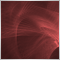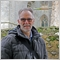# Simple question for passing data from Indicator to my Expert Advisor19

Hi everyone!

This has been asked many times and I've read so much on it, but I'm still not accomplishing what I want (which should be very simple).

I have an Indicator, and all I want is to grab the value of a variable which could be a 0 or a 1. That's it.

DISCLOSURE: I'm new to MetaTrader and coding in it.

```#property indicator_separate_window
#property indicator_buffers 2
#property indicator_plots   1
#property indicator_label1  "TrendFlex"
#property indicator_type1   DRAW_COLOR_LINE
#property indicator_color1  clrDodgerBlue,clrCoral
#property indicator_width1  2

//
//---
//

input int inpFastPeriod = 20; // Fast trend-flex period
input int inpSlowPeriod = 50; // Slow trend-flex period
double  val[],valc[];

//------------------------------------------------------------------
//
//------------------------------------------------------------------
//
//
//

int OnInit()
{
SetIndexBuffer(0,val   ,INDICATOR_DATA);
SetIndexBuffer(1,valc  ,INDICATOR_COLOR_INDEX);

iTfFast.OnInit(inpFastPeriod);
iTfSlow.OnInit(inpSlowPeriod);

//
//
//

IndicatorSetString(INDICATOR_SHORTNAME,"Meme TrendFlex ("+(string)inpFastPeriod+","+(string)inpSlowPeriod+")");
return(INIT_SUCCEEDED);
}

//------------------------------------------------------------------
//
//------------------------------------------------------------------
//
//
//
//

int OnCalculate(const int rates_total,
const int prev_calculated,
const datetime &time[],
const double &open[],
const double &high[],
const double &low[],
const double &close[],
const long &tick_volume[],
const long &volume[],
{
int limit = MathMax(prev_calculated-1,0);
for(int i=limit; i<rates_total && !_StopFlag; i++)
{
double _price = (i>0 ? (close[i]+close[i-1])/2 : close[i]);
val[i]  = iTfFast.OnCalculate(_price,i,rates_total)-iTfSlow.OnCalculate(_price,i,rates_total);
valc[i] = (i>0) ? val[i]>val[i-1] ? 0 :  val[i]<val[i-1] ? 1 : 0 : 0;
}
return(rates_total);
}

//------------------------------------------------------------------
//
//------------------------------------------------------------------
//
//
//

class CTrendFlex
{
private :
double m_c1;
double m_c2;
double m_c3;
struct sWorkStruct
{
double value;
double ssm;
double sum;
double ms;
};
sWorkStruct m_array[];
int         m_arraySize;
int         m_period;

public :
CTrendFlex() : m_c1(1), m_c2(1), m_c3(1), m_arraySize(-1) {  }
~CTrendFlex()                                              {  }

//
//---
//

void OnInit(int period)
{
m_period = (period>1) ? period : 1;

double a1 = MathExp(-1.414*M_PI/m_period);
double b1 = 2.0*a1*MathCos(1.414*M_PI/m_period);
m_c2 = b1;
m_c3 = -a1*a1;
m_c1 = 1.0 - m_c2 - m_c3;
}
double OnCalculate(double value, int i, int bars)
{
if (m_arraySize<bars) m_arraySize=ArrayResize(m_array,bars+500);

//
//
//

m_array[i].value = value;
if (i>1)
m_array[i].ssm = m_c1*(m_array[i].value+m_array[i-1].value)/2.0 + m_c2*m_array[i-1].ssm + m_c3*m_array[i-2].ssm;
else    m_array[i].ssm = value;
if (i>m_period)
m_array[i].sum = m_array[i-1].sum + m_array[i].ssm - m_array[i-m_period].ssm;
else
{
m_array[i].sum = m_array[i].ssm;
for (int k=1; k<m_period && (i-k)>=0; k++) m_array[i].sum += m_array[i-k].ssm;
}

//
//
//

double sum   = m_period*m_array[i].ssm-m_array[i].sum;
sum  /= m_period;

m_array[i].ms = (i>0) ? 0.04 * sum*sum+0.96*m_array[i-1].ms : 0;
return (m_array[i].ms!=0 ? sum/MathSqrt(m_array[i].ms) : 0);
}
};
CTrendFlex iTfSlow,iTfFast;```

And this is what I'm typing within my Expert Advisor file to get that value (I'm using MetaTrader 5):

`double MyValue = iCustom(NULL,0,"TrendFlex",1,0);`

Any help would be greatly appreciated.

Thank you all!5046

carloscordon82:

Hi everyone!

This has been asked many times and I've read so much on it, but I'm still not accomplishing what I want (which should be very simple).

I have an Indicator, and all I want is to grab the value of a variable which could be a 0 or a 1. That's it.

DISCLOSURE: I'm new to MetaTrader and coding in it.

And this is what I'm typing within my Expert Advisor file to get that value (I'm using MetaTrader 5):

Any help would be greatly appreciated.

Thank you all!

look up icustom for mql5.  You need to get handle in oninit first5

hi,what's the meaning of m after eurusd-m?2249

Dear Metaeditor user,

I also new but what I know is that the return value of the iCustom function is an Int, not an double and can not be a double.

the result is a handle, that you can use to work with the personal written indicator.

You will need additional code, lot of additional code to get the values from the indicator using a handle.

Succes23446

mehdi5396: what's the meaning of m after eurusd-m?

Broker's use a variety of naming patterns: EURUSD, EURUSDc, EURUSDct, EURUSDecn, EURUSDi, EURUSDm, EURUSDpro, EURUSDt, "EUR.USD", "EUR/USD", "EURUSD!", "EURUSD#", "EURUSD.", "EURUSD..", "EURUSD.c", "EURUSD.cfx", "EURUSD.G", "EURUSD.SBe", "EURUSD.stp", "EURUSD+", "EURUSD-5", "EURUSD-m", "EURUSD-sb", etc.

If the pattern your broker uses doesn't match the pattern of your signal provider's broker, you can't copy the signal.40759

William Roeder: